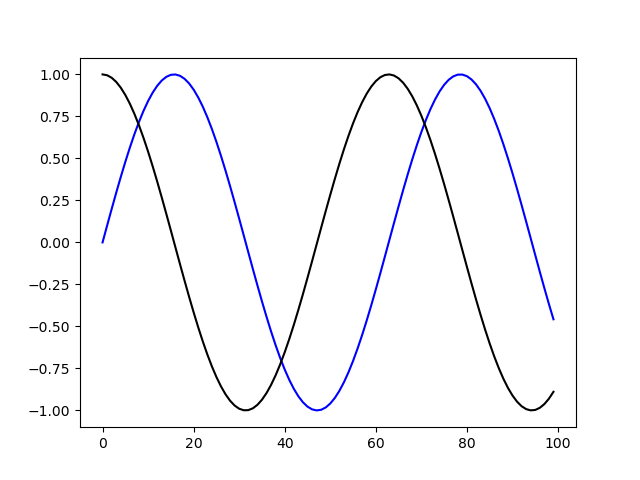# Add Legend to Figure in MatplotlibAdd Legend to Figure in Matplotlib. In this article, we'll take a look at how to add a legend to a Matplotlib plot.

#### Introduction

Matplotlib is one of the most widely used data visualization libraries in Python. Typically, when visualizing more than one variable, you'll want to add a legend to the plot, explaining what each variable represents.

In this article, we'll take a look at how to add a legend to a Matplotlib plot.

#### Creating a Plot

Let's first create a simple plot with two variables:

``````import matplotlib.pyplot as plt
import numpy as np

fig, ax = plt.subplots()

x = np.arange(0, 10, 0.1)
y = np.sin(x)
z = np.cos(x)

ax.plot(y, color='blue')
ax.plot(z, color='black')

plt.show()``````

Here, we've plotted a sine function, starting at `0` and ending at `10` with a step of `0.1`, as well as a cosine function in the same interval and step. Running this code yields:Now, it would be very useful to label these and add a legend so that someone who didn't write this code can more easily discern which is which.

## Matplotlib Tutorial | Python Matplotlib Library

Matplotlib Tutorial. Matplotlib is one of the most popular Python packages used for data visualization. It is a cross-platform library for making 2D plots from data in arrays. It provides an object-oriented API that helps in embedding plots in applications using Python GUI toolkits such as PyQt, WxPythonotTkinter.

## Python Matplotlib Introduction & Installation

This is our first tutorial in Python Matplotlib, in this tutorial we are going to learn about Python Matplotlib Introduction & Installation.

## Python Matplotlib How to Plot Points

In this article we are going to learn Matplotlib How to Plot Points. also we can call it scatter plot.  you

## Python Matplotlib Plotting Barchart - Code Loop

A vertical bar chart is sometimes called a column chart. you can read the previous articles for Python Matplotlib in the below links.

## Python Tricks Every Developer Should Know

In this tutorial, you’re going to learn a variety of Python tricks that you can use to write your Python code in a more readable and efficient way like a pro.